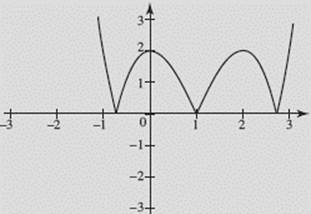﻿ ﻿SPECIAL FUNCTIONS - Functions - Calculus AB and Calculus BC

## CHAPTER 1 Functions

### B. SPECIAL FUNCTIONS

The absolute-value function f (x) = |x| and the greatest-integer function g(x) = [x] are sketched in Figure N1–3.FIGURE N1–3

EXAMPLE 8

A function f is defined on the interval [−2, 2] and has the graph shown in Figure N1–4.

(a) Sketch the graph of y = |f (x)|.

(b) Sketch the graph of y = f (|x|).

(c) Sketch the graph of y = − f (x).

(d) Sketch the graph of y = f (−x).FIGURE N1–4

SOLUTIONS: The graphs are shown in Figures N1–4a through N1–4d.FIGURE N1–4aFIGURE N1–4bFIGURE N1–4cFIGURE N1–4d

Note that graph (c) of y = − f (x) is the reflection of y = f (x) in the x-axis, whereas graph (d) of y = f (−x) is the reflection of y = f (x) in the y-axis. How do the graphs of |f (x)| and f (|x|) compare with the graph of f (x)?

EXAMPLE 9

Let f (x) = x3 − 3 x2 + 2. Graph the following functions on your calculator in the window [−3,3] × [−3,3]: (a) y = f (x); (b) y = |f (x)|; (c) y = f (|x|).

SOLUTIONS:

(a) y = f (x)

See Figure N1–5a.FIGURE N1–5a

(b) y = |f (x)|

See Figure N1–5b.FIGURE N1–5b

(c) y = f (|x|)

See Figure N1–5c.FIGURE N1–5c

Note how the graphs for (b) and (c) compare with the graph for (a).

﻿